JEE  >  Test: Complex Numbers

# Test: Complex Numbers

Test Description

## 30 Questions MCQ Test Mathematics (Maths) Class 11 | Test: Complex Numbers

Test: Complex Numbers for JEE 2023 is part of Mathematics (Maths) Class 11 preparation. The Test: Complex Numbers questions and answers have been prepared according to the JEE exam syllabus.The Test: Complex Numbers MCQs are made for JEE 2023 Exam. Find important definitions, questions, notes, meanings, examples, exercises, MCQs and online tests for Test: Complex Numbers below.
Solutions of Test: Complex Numbers questions in English are available as part of our Mathematics (Maths) Class 11 for JEE & Test: Complex Numbers solutions in Hindi for Mathematics (Maths) Class 11 course. Download more important topics, notes, lectures and mock test series for JEE Exam by signing up for free. Attempt Test: Complex Numbers | 30 questions in 60 minutes | Mock test for JEE preparation | Free important questions MCQ to study Mathematics (Maths) Class 11 for JEE Exam | Download free PDF with solutions
 1 Crore+ students have signed up on EduRev. Have you?
Test: Complex Numbers - Question 1

### Number of values `p' for which the equation (p2 – 3p + 2) x2 – (p2 – 5p + 4)x + p – p2 = 0 possess more than two roots, is

Detailed Solution for Test: Complex Numbers - Question 1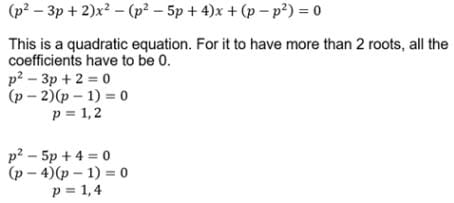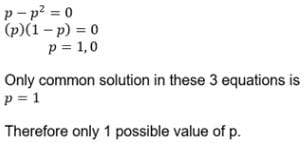Test: Complex Numbers - Question 2

### The roots of the equation (b – c) x2 + (c – a) x + (a – b) = 0 are

Detailed Solution for Test: Complex Numbers - Question 2

Clearly, x = 1 is a root of the given equation.
Let, alpha, beta are the roots ; alpha = 1
We know , product of the roots :-
Alpha * Beta = a-b / b-c
1* ( beta) = a-b / b- c
Beta = a-b/ b- c
Therefore, roots are :- ( a-b/b-c, 1)

Test: Complex Numbers - Question 3

### If a, b are the roots of quadratic equation x2 + px + q = 0 and g, d are the roots of x2 + px – r = 0, then (a – g) . (a - d) is equal to

Detailed Solution for Test: Complex Numbers - Question 3

x2+px+q=0 have roots a,b

a=b=-p  --(1)

ab = q  --(2)

a2+pa+q=0  --(3)

x2+px−r=0 have roots g,d

g+d=-p  --(4)

gd = -r  --(5)

(a-g)(a-d) = a2−(g−d)a+gd

= a2+pa−r = -q-r =-(q+r)  --from (3)

Test: Complex Numbers - Question 4

Two real numbers a & b are such that a + b = 3 & |a - b| = 4, then a & b are the roots of the quadratic equation

Detailed Solution for Test: Complex Numbers - Question 4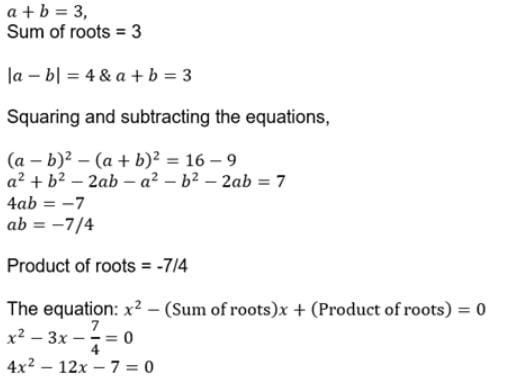Test: Complex Numbers - Question 5

If a, b, c are integers and b2 = 4(ac + 5d2), d ∈ N, then roots of the equation ax2 + bx + c = 0 are

Detailed Solution for Test: Complex Numbers - Question 5

b2 = 4(ac + 5d2)
D = b2 − 4ac
= 4(ac + 5d2) − 4ac
= 4ac + 20d2 − 4ac
= 20d2
Now, x = (−b ± √D)/2a
=(− b ± √20d2)/2a
=(− b ± 2√5d)/2a
Therefore, roots of the given quadratic equation are irrational.

Test: Complex Numbers - Question 6

Let a, b and c are real numbers such that 4a + 2b + c = 0 and ab > 0. Then the equation ax2 + bx + c = 0 has

Detailed Solution for Test: Complex Numbers - Question 6

Put  x=2  in  the  LHS  of  the  quadratic  equation
We  get,  4a  + 2b + c = 0
Hence  x=2  is  a  root  of  the  equation. (Real roots)

Test: Complex Numbers - Question 7

Which of the following graph represents expression f(x) = ax2 + bx + c (a < 0) when a > 0, b < 0 & c < 0 ?

Detailed Solution for Test: Complex Numbers - Question 7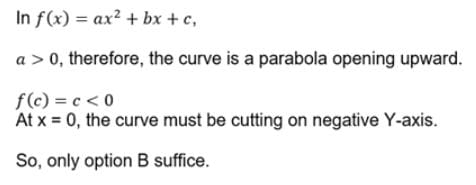Test: Complex Numbers - Question 8

The expression y = ax2 + bx + c has always the same sign as of `a' if

Detailed Solution for Test: Complex Numbers - Question 8

For the quadratic curve to always have the same sign, it must never cut the X-axis, i    i.e. it must not have any real roots.
So, b2 – 4ac < 0
4ac > b2

Test: Complex Numbers - Question 9

If a, b ∈ R, a < 0 and the quadratic equation ax2 – bx + 1 = 0 has imaginary roots then a + b + 1 is

Detailed Solution for Test: Complex Numbers - Question 9

f(x) = ax2 - bx + c
f(0) = 1 (positive)
That is, f(x) = ax2 - bx + 1 = 0
Therefore f(x) has imaginary roots
f(-1) = a+b+c > 1 (Hence positive)

Test: Complex Numbers - Question 10

If a and b are the non–zero distinct roots of x2 + ax + b = 0, then the least value of x2 + ax + b is

Detailed Solution for Test: Complex Numbers - Question 10

Solution :- a and b of x^2 + ax + b = 0

Sum of roots : a + b = -a, 2a + b = 0

Product of roots : ab = b, b(a-1)

Minimum value of x^2 + ax + b at x =-a/2

We get a = -9/4

Test: Complex Numbers - Question 11

If y = –2x2 – 6x + 9, then

Detailed Solution for Test: Complex Numbers - Question 11

y = -2x2 - 6x + 9
dy/dx = -4x - 6
Put dy/dx = 0
-4x - 6 = 0
x = -3/2   = 1.5
d2y/dx2 = -4 < 0  (Maximum point)
Max value = -2(-1.5)2 - 6(-1.5) + 9
= 13.5
Max value of x = -1.5

Test: Complex Numbers - Question 12

The number of the integer solutions of x2 + 9 < (x + 3)2 < 8x + 25 is

Detailed Solution for Test: Complex Numbers - Question 12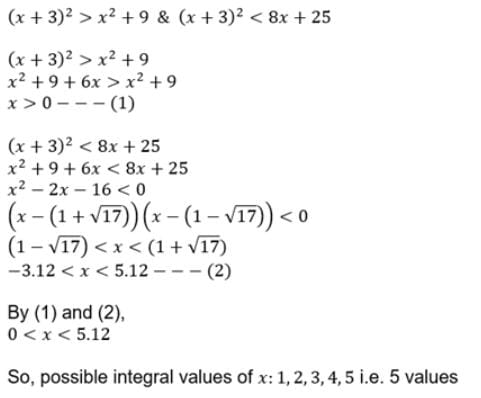Test: Complex Numbers - Question 13

If both roots of the quadratic equation (2 – x) (x + 1) = p are distinct & positive, then p must lie in the interval

Detailed Solution for Test: Complex Numbers - Question 13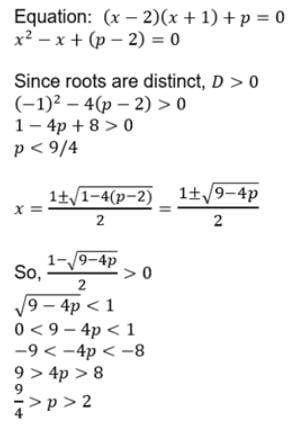Test: Complex Numbers - Question 14

The equatiobn πx = –2x2 + 6x – 9 has

Detailed Solution for Test: Complex Numbers - Question 14

The RHS of the expression has a<0 which means the graph will lie below the x-axis and πx lies above the x-axis.Therefore,no solution.

Test: Complex Numbers - Question 15

Let a > 0, b > 0 and c > 0. Then both the roots of the equation ax2 + bx + c = 0

Detailed Solution for Test: Complex Numbers - Question 15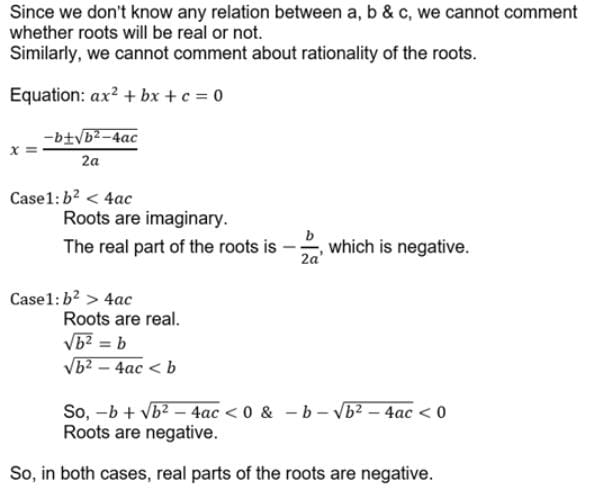Test: Complex Numbers - Question 16

The set of all solutions of the inequality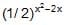< 1/4 contains the set

Detailed Solution for Test: Complex Numbers - Question 16

(1/2)(x2 - 2x) < (1/4)
(1/2)(x2 - 2x) < (1/2)2
x2 − 2x > 2......(as after multiplicative inverse sign of inequality changes)
x2 − 2x − 2 > 0
x2  -  2x + 1 - 3  >
(x-1)2  - 3  > 0
(x-1)2  > 3
So for the above to hold good both the expression must be positive or both must be negative. After finding the solution the range of the solution will be
either x > 3
(3,¥)

Test: Complex Numbers - Question 17

Consider y =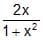, where x is real, then the range of expression y2 + y – 2 is

Detailed Solution for Test: Complex Numbers - Question 17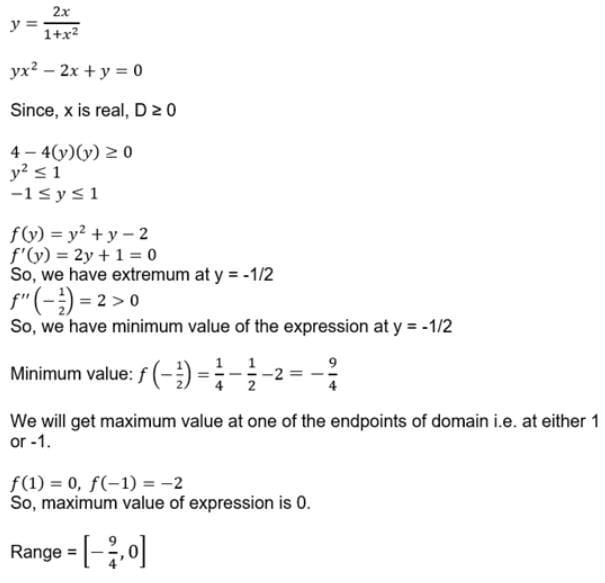Test: Complex Numbers - Question 18

The values of k, for which the equation x2 + 2(k – 1) x + k + 5 = 0 possess atleast one positive root, are

Detailed Solution for Test: Complex Numbers - Question 18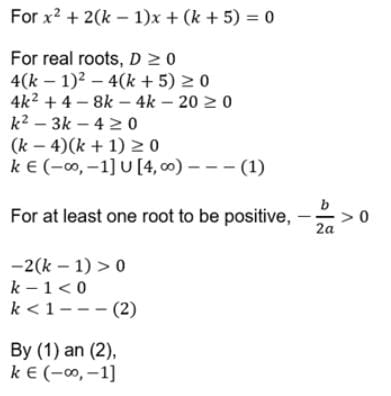Test: Complex Numbers - Question 19

If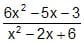<= 4, then least and the highest values of 4x2 are

Detailed Solution for Test: Complex Numbers - Question 19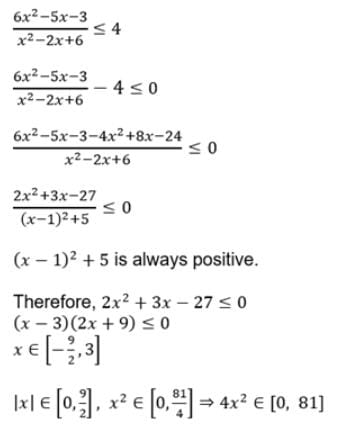Test: Complex Numbers - Question 20

If coefficients of the equation ax2 + bx + c = 0, a < 0 are real and roots of the equation are non–real complex and a + c + b < 0, then

Detailed Solution for Test: Complex Numbers - Question 20

Puting x=-1
a-b+c
but
a+c<b or a+c-b<0
hence f(x)<0 for all real values of x
therefore
putting x=-2
we get f(X)=4a+c-2b<0
or 4a+c<2b

Test: Complex Numbers - Question 21

If the roots of the equation x2 + 2ax + b = 0 are real and distinct and they differ by at most 2m, then b lies in the interval

Detailed Solution for Test: Complex Numbers - Question 21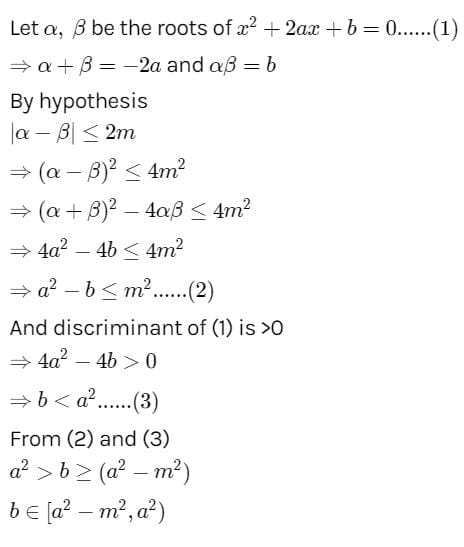Test: Complex Numbers - Question 22

If the roots of the quadratic equation x2 + px + q = 0 are tan 30º and tan 15º respectively, then the value of 2 + q – p is

Test: Complex Numbers - Question 23

If (1 – p) is root of quadratic equation x2 + px + (1 – p) = 0, then its roots are

Detailed Solution for Test: Complex Numbers - Question 23

As (1 – p) is root of the equation: x2 + px + (1 – p) = 0

(1 – p)2 + p(1 – p) + (1 – p) = 0

(1 – p)[1 – p + p + 1] = 0

(1 – p) = 0

p = 1

Therefore, given equation now becomes

x2 + x = 0

x(x + 1) = 0

x = 0, -1

Test: Complex Numbers - Question 24

The values of x and y besides y can satisfy the equation (x, y ∈ real numbers) x2– xy + y2 – 4x – 4y + 16 = 0

Test: Complex Numbers - Question 25

For what value of a and b the equation x4 – 4x3 + ax2 + bx + 1 = 0 has four real positive roots ?

Detailed Solution for Test: Complex Numbers - Question 25

Since all roots are positive,
coeff. of x shld be negatuve i.e., b is negative
let roots be m(>0), n(>0), p(>0), q(>0)
m+n+p+q = 4
mn+np+pq+mp+mq+nq = a
mnp+mnq+npq+mpq = -b
mnpq = 1
For the objective case,
put m=n=p=q =1
so a = 1+1+1+1+1+1 = 6
-b = 1+1+1+1 =4
so, a = 6 and b = -4

Test: Complex Numbers - Question 26

If a, b are roots of the equation ax2 + bx + c = 0, then the value of a3 + b3 is

Test: Complex Numbers - Question 27

If z1 = 2 + i, z2 = 1 + 3i, then Re ( z1 - z2) =

Detailed Solution for Test: Complex Numbers - Question 27

The numbers in the questions are not very clear.
z1 = 2 + i
z2 = 1+3i
z1 – z2 = (2 – 1) + i (1 – 3)
= 1 – 2i

Test: Complex Numbers - Question 28

If a, b are roots of the equation ax2 + bx + c = 0 then the equation whose roots are 2a + 3b and 3a + 2b is

Test: Complex Numbers - Question 29

If S is the set of all real x such that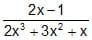is positive, then S contains

Detailed Solution for Test: Complex Numbers - Question 29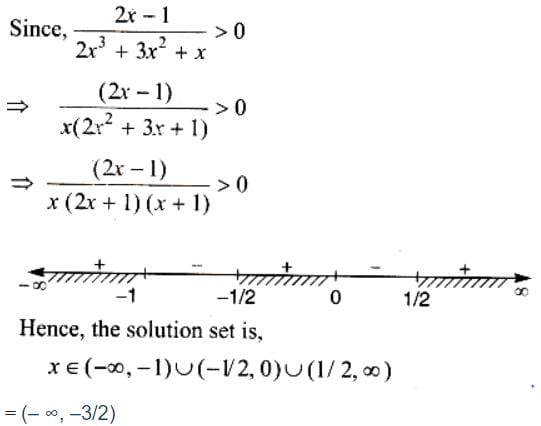Test: Complex Numbers - Question 30

If the roots of the equation x2 - 5x + 16 = 0 are a, b and the roots of the equation x2 + px + q = 0 are (a2 + b2) and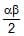, then-

## Mathematics (Maths) Class 11

156 videos|176 docs|132 tests
Information about Test: Complex Numbers Page
In this test you can find the Exam questions for Test: Complex Numbers solved & explained in the simplest way possible. Besides giving Questions and answers for Test: Complex Numbers , EduRev gives you an ample number of Online tests for practice

## Mathematics (Maths) Class 11

156 videos|176 docs|132 tests(Scan QR code)